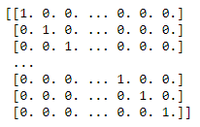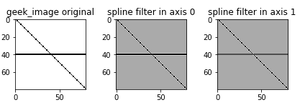Related Articles

# Python SciPy – ndimage.spline_filter1d() function

• Last Updated : 21 Jul, 2021

This method is used to calculate a 1-D spline filter along the given axis. These are filtered by a spline filter.

Syntax: scipy.ndimage.spline_filter1d(input, order=3, axis=-1, output=<class ‘numpy.float64’>)

Parameters

input: array_like – The input array

order: int – The order of the spline, default is 3.

axis: int,  – The axis along which the spline filter is applied. Default is the last axis.

output: ndarray  – The array in which to place the output, or the dtype of the returned array. Default is numpy.float64.

Example 1:

## Python3

 `# importing spline filter with one dimension.``from` `scipy.ndimage ``import` `spline_filter1d` `# importing matplot library for visualization``import` `matplotlib.pyplot as plt` `# importing munpy module``import` `numpy as np` `# creating an image``geek_image ``=` `np.eye(``80``)` `# returns an image array format``geek_image[``40``, :] ``=` `1.0``print``(geek_image)`

Output:Example 2:

## Python3

 `# importing spline filter with one dimension.``from` `scipy.ndimage ``import` `spline_filter1d` `# importing matplot library for visualization``import` `matplotlib.pyplot as plt` `# importing munpy module``import` `numpy as np` `# creating an image``geek_image ``=` `np.eye(``80``)` `geek_image[``40``, :] ``=` `1.0` `# in axis=0``axis_0 ``=` `spline_filter1d(geek_image, axis``=``0``)` `# in axis=1``axis_1 ``=` `spline_filter1d(geek_image, axis``=``1``)` `f, ax ``=` `plt.subplots(``1``, ``3``, sharex``=``True``)` `for` `ind, data ``in` `enumerate``([[geek_image, ``"geek_image original"``],``                            ``[axis_0, ``"spline filter in axis 0"``],``                            ``[axis_1, ``"spline filter in axis 1"``]]):``    ``ax[ind].imshow(data[``0``], cmap``=``'gray_r'``)``    ` `    ``# giving title``    ``ax[ind].set_title(data[``1``])` `    ``# orientation layout of our image``plt.tight_layout()` `# to show image``plt.show()`

Output:Attention geek! Strengthen your foundations with the Python Programming Foundation Course and learn the basics.

To begin with, your interview preparations Enhance your Data Structures concepts with the Python DS Course. And to begin with your Machine Learning Journey, join the Machine Learning – Basic Level Course

My Personal Notes arrow_drop_up